## Tuesday, March 27, 2012

### No holes

In http://www.or-exchange.com/questions/5105/transforming-constraint-with-max-function a question arise how to forbid “holes” in a series of ones. E.g.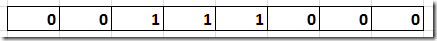is allowed, but the following is not allowed: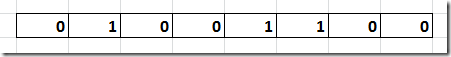Actually this is a well-known problem in machine scheduling. E.g. often we want to limit the number of start-ups of a generator (e.g. to limit wear and tear).

I believe the suggested solution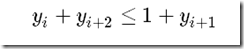is not 100% correct as it will only forbid holes of length 1. Of course we can repeat this constraint for holes of size 2,3,….

There is another approach for this: count the number of time we see a startup. This can look like:I.e. a startup happens of we go from 0 to 1.

Notes:

• s(i) is automatically integer so we can relax if that is beneficial.
• s(i) is not equal to a startup: it is a bound. Even if all y’s are zero, there may be a nonzero s(i).
• always pay attention to the boundaries, e.g. what happens if machine is started up in first period.

### LocalSolver

This looks like an interesting tool that can be very effective on certain model classes with 0-1 variables.

I believe this is a local search tool with a simulated annealing framework. From what I understand this method really wants to evaluate many solutions as quickly as possible. The method does not like real hard constraints (i.e. preferably constraints go into the objective with an appropriate cost or penalty when violated). In the words of the above paper: “LocalSolver is not designed for solving hardly-constrained optimization problems”.

I suspect the graph shown in http://localsolver.com/images/content/complexity_curves.png is only representative for problems that are suited for this LS approach (so may be no need yet to throw away our MIP and CP solvers).

This also caught my eye:

## Monday, March 26, 2012

### Time-indexed scheduling model

In the model I am working on we use a time indexed binary variable to model the schedule:

 variables    makespan    x(j,t) '=1 if job j starts in period t'    s(j) 'start of job'    z    'elastic infeasibility' ; binary variable x(j,t); positive variable z;

When I solved this model for 1000 seconds, I observed that most of the action takes place in the first few nodes. I was thinking that the following simple algorithm may work to get better improvements:

1. Solve for a shorter time with initial planning horizon giving us a makespan<horizon.
2. Reduce planning horizon to the makespan, and resolve. This model is now smaller, and with MIPSTART we can quickly branch towards the last found solution.
3. Repeat step 2 until out of time.

The loop looks like:

 dt.optfile=1; dt.reslim=200; set iter/iter1*iter5/; loop(iter,   solve dt minimizing makespan using mip;   x.fx(j,t)\$(tval(t)+duration(j)>makespan.l)=0;   z.fx\$(z.l<0.01) = 0;   dt.optfile=2; );

This looks like a reasonable approach: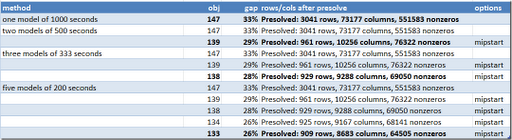At least for this case it is better to solve 5 models for 200 seconds than one model for 1,000 seconds. Notice that we reduce the size of the problem in each cycle.

## Friday, March 23, 2012

### ODBC 32/64 bit

Related to http://support.microsoft.com/kb/942976, if you call Cscript from a 64 bit environment you may end up in a 64 bit ODBC world.

E.g. when calling from 64 bit GAMS using

\$call cscript script.vbs

I encountered:

 Microsoft (R) Windows Script Host Version 5.8 Copyright (C) Microsoft Corporation. All rights reserved. Provider:MSDASQL  Version:6.1  C:\projects\erwin\script.vbs(10, 1) Microsoft OLE DB Provider for ODBC Drivers: [Microsoft][ODBC Driver Manager] Data source name not found and no default driver specified

To get into the 32 bit world use:

\$call \windows\syswow64\cscript script.vbs

Now we get more normal behavior:

### Writing solutions to MySQL

I was looking at some code from a client where solution records are stored in a MySQL database. They use standard SQL INSERT statements to do this. Even for small number of solution records this is quite slow. I suggest a few simple improvements. E.g. MySQL has an interesting SQL INSERT extension where you can add multiple VALUEs to a single INSERT statement, like:

INSERT INTO mytable (a,b,c) VALUES (1,2,3),(4,5,6),(7,8,9)

In addition I surrounded the INSERTs by a transaction, which will increase the performance even more (there will be only one commit). For the fastest performance a bulk insert can be done using the LOAD DATA INFILE statement which can load a text file directly into a table.

For n=1,000 solution records I saw:

 Method Time for 1,000 solution points Note (1) INSERT 41 seconds this was the original setup: 12,000 inserts (2) INSERT multiple values 7 seconds 2,000 inserts (2)+transactions 1.2 seconds 2,000 inserts LOAD DATA INFILE 0.3 seconds 2 statements to load 2 csv files

Some optimization models generate a ton of solution results, and in those cases it may be useful to spend a little bit of time on deciding how to store solutions in a database.

## Wednesday, March 21, 2012

### Elastic formulation of scheduling problems

When deploying optimization models in a production environment, the problem of dealing with infeasibilities is always a nasty complication. If the model is infeasible (either because the equations are really have no feasible solution, or we did not find a feasible solution in the allocated time), it is difficult to provide any useful feedback.

One way to handle this situation is to formulate an elastic model where we allow extra resources to be “purchased” at a price. This will make the model feasible so at least we can report something back. In addition this may give good feedback about a cheap way to alleviate resource bottlenecks in the system.

An additional advantage is that an elastic formulation may actually work better in practice in finding good solutions. MIP solvers often perform best when they produce good integer solutions early on during the search. Here is a example. In this scheduling problem using a standard formulation no solution was found in the first 1000 seconds. When we used an elastic formulation we found a number of good solutions in that time. In the beginning the solutions required extra resources, but within about a hundred seconds good solutions without penalties were produced.

So the irony is that we used an elastic formulation to be able to make some sense out of infeasible solutions, but while doing that actually were able to produce feasible solutions for the original problem.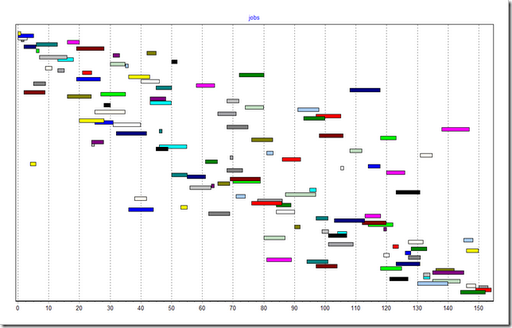## Wednesday, March 14, 2012

### GAMS GDX performance

Reading and writing GDX files is very fast in GAMS. However I encountered some relative slowdown in some cases. These all involved huge GDX files (something like 1.7 GB). With a small example I could reproduce this.

 Model A set i /i1*i400/; alias (i,j,k); parameter p(i,j,k); p(i,j,k) = uniform(0,1); execute_unload "1.gdx"; With this model we can generate a large test file. The parameter p has 64 million entries. The file size is 0.6 GB. Model B set i /i1/; parameter p(i,*,*); \$gdxin 1.gdx \$load p This model reads a slice of the data. It takes 13 seconds, which is longer than I expected. Note that I usually recommend to use \$loaddc, but here the use of \$load is intentional. Model C set i /i1*i400/; parameter p(i,*,*); \$gdxin 1.gdx \$load p This model reads the whole thing in 6 seconds. Hence Model B should take less than 6 seconds. Model D parameter p(*,*,*); \$gdxin 1.gdx \$load p This is almost the same as Model C but needs 11 seconds. Should run as fast as Model C.

I expected Model C and D to perform the same and Model B to be significantly faster. For Model B the GDX routines will still do what is sometimes called a complete table scan, but as we can skip a lot of records this still should be faster than loading the whole thing.

I reported in the above table total times. In some cases we lose time somewhere in the model. E.g. if we run Model B with option PROFILE=1 we see:

 1  set i /i1/;    2  parameter p(i,*,*); GDXIN   C:\projects\tmp\load\1.gdx --- LOAD  p = 4:p ----      4 \$load                    6.724     6.724 SECS      8 Mb COMPILATION TIME     =       13.588 SECONDS      8 Mb  WEX237-237 Aug 23, 2011

I suspect the \$load timing is not correct as we don’t do anything else in the model.

Update: the reason for the slow \$load performance of model B has been fixed in the GDXIO library. I assume this fix is too late for 23.8.

## Monday, March 12, 2012

### Exporting Excel Tables

GDXXRW is a flexible GAMS tool to read and write Excel files. Here is an example output sheet:

A few things I would like to be done better such that the resulting tables look a little bit less basic. GDXXRW has some facilities to deal with formatting (such as Merge), but these really only work well with static tables so that a predefined format can be used. In my case the size of the table is not known in advance. With a JavaScript based script I clean up all the tables (approx. 60 of them), so the results look like:

As these tables will be generated by a Web based front-end, the whole thing should run automatically and without user intervention.

Note: the warning about numbers being formatted as strings is related to set elements (years in this case) that look like numbers. This warning can be disabled with an Excel option.

## Wednesday, March 7, 2012

### GAMS+GDXXRW under IIS 7 Webserver

Running a GAMS job using GDXXRW from IIS 7 webserver can give several errors.

`Cannot create an instance of Excel: Error = Access is deniedCheck that Excel is installed correctly.In case you use the "Click-to-Run" version of Excel, uninstallthis version and install the regular version.`

This can be fixed by giving the group IIS_IUSRS permission to use COM automation (via dcomcnfg.exe).

`**** Problem opening Excel file: Microsoft Office Excel cannot open or save any more documents because there is not enough available memory or disk space. • To make more memory available, close workbooks or programs you no longer need. • To free disk space, delete files you no longer need from the disk you are saving to`

This can be solved by adding a Desktop directory to C:\Windows\SysWOW64\config\systemprofile. See: http://social.msdn.microsoft.com/Forums/en-US/innovateonoffice/thread/b81a3c4e-62db-488b-af06-44421818ef91

## Tuesday, March 6, 2012

### Checking Math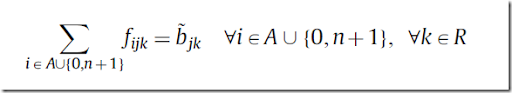A system like LaTeX will not check these equations for correctness. Obviously also human readers have troubles spotting these errors (otherwise this would not have slipped through the review process). However if the authors would have run this model through a modeling language like AMPL or GAMS, this error would yield syntax errors.

Of course such a mistake can still happen when translating AMPL or GAMS to LaTeX by hand. Now and then I see requests for some tool to convert AMPL/GAMS equations to LaTeX automatically.

## Saturday, March 3, 2012

### RSPSP

Here is an example of a simple resource-constrained project scheduling problem formulation as presented in a computational study:I probably would write this as:This can save us a few non-zeros in the problem. In general if there are repetitive structures in the model, you should think about introducing extra variables and constraints. So I would write the model equations in GAMS like:

calcstart(j)..
y(j) =e=
sum
(t, tval(t)*x(j,t));

objective..
makespan =e=
sum
(last, y(last));

precedence(prec(i,j))..
y(j) =g= y(i) + duration(i);

resources(r,t)..

sum
(overlap(j,t,tstart),usage(j,r)*x(j,tstart)) =l= available(r);

onestart(j)..

sum
(t, x(j,t)) =e= 1;

Notes:
• The set start is a singleton set. Looks like a summation in the objective, but it really is just picking the correct element.
• The set overlap is constructed to simplify equation (5). In general equations are more difficult to debug than sets. They can only be looked at in the listing file after you have a working model (the equation listing is only visible when . Sets can more easily be inspected (using a display statement or through the gdx viewer). So it makes sense to introduce extra sets to make the equations as simple as possible.

The paper presenting this model (here) makes -- in my opinion -- a mistake by only considering the number of variables and equations as a measure of the size of a particular model formulation. The number of nonzero elements should also be considered, and sometimes we are happy to add more variables and equations if this reduces the number of nonzero elements in a significant way.